# Interactive Sample CLAST Math Self Tests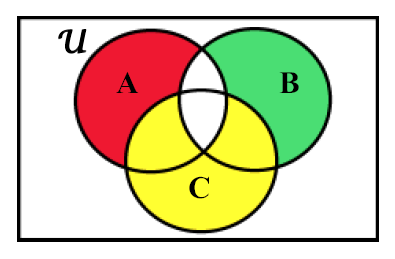The Math Section of the College Level Academic Skills Testing program consists of 56 objectives divided into five subtests: Arithmetic, Algebra, Geometry, Statistics, and Logical Reasoning. Each question on the Math Sample Exams covers one of the 56 objectives (a list of the objectives appear below). These 56 questions should be completed in 90 minutes. A timer has been placed at the top of each test for you to keep track of the amount of time it takes you to complete the test. (The timer will not stop your test after 90 minutes expires)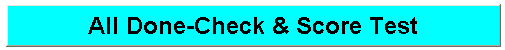When you have finished the sample test, press the "All Done Check & Score Test" button. This will grade the test as well as divide the grade into five subtest grades. The Pop-up window will show your correct and incorrect responses. You may print this results window from the menubar of the popup window.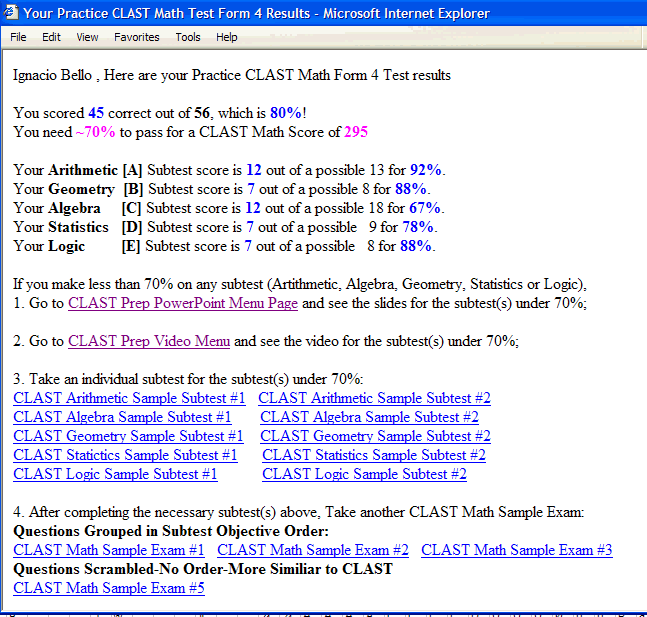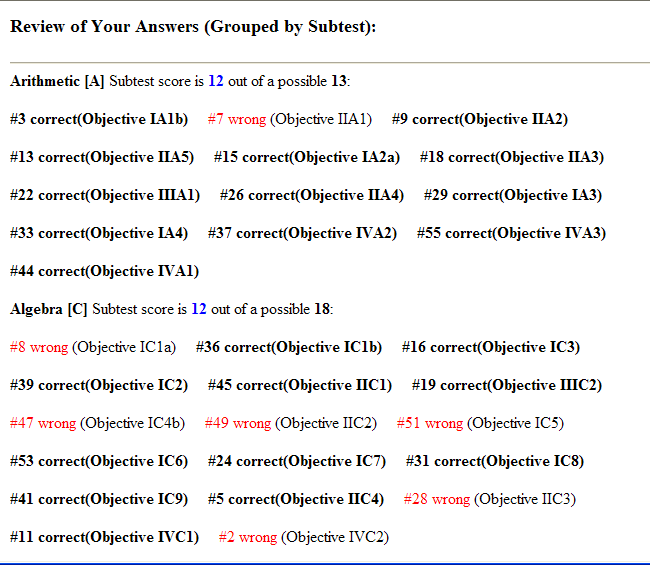After checking the test and correcting your answers, you may press the "Submit Answers" button. Your self-test is confidential to you and only you. This "Submit Answers" button will send only you an email with a record of your testing: your responses, the correct answers, the subtest scores, the total test score, and the time elapsed to complete the test.

A diagnostic assessment (Prescription) will also be included in this email with links to subtest powerpoint slides, subtest online videos, sample subtests, and additional 56 question practice tests.

No records of these tests are kept by anyone: not USF, not the server manager, not Ignacio Bello, nor the author of this program. The only record is the email sent to you.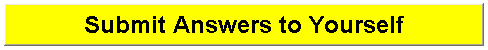After pressing the submit button all responses are cleared.

These five following Interactive 56 question Sample Exams are from the CLAST math generator prepared by Ignacio Bello:

### Questions Grouped in Subtest Objective Order

CLAST Math Sample Exam #1
CLAST Math Sample Exam #2
CLAST Math Sample Exam #3

### Questions Scrambled-No Order Nor Subtest Grouping

CLAST Math Sample Exam #4
CLAST Math Sample Exam #5
Please note in test #4 and #5 above, all questions are scrambled

In addition to the five tests above, we have the following practice Subtests, PowerPoint Slides, and online videos for student having specific subtest problems:

### Arithmetic Skills Interactive Practice Subtests:

CLAST Arithmetic Sample Subtest #1
CLAST Arithmetic Sample Subtest #2

Slide Presentation #1: CLAST Prep Arithmetic PowerPoint
Slide Presentation #2: CLAST Prep Arithmetic PowerPoint
VIDEO #1 CLAST Prep: Arithmetic (1 hour Streaming Video) (42k-100k for all Connections)

### Algebra Skills Subtests:

CLAST Algebra Sample Subtest #1
CLAST Algebra Sample Subtest #2

Slide Presentation #1: CLAST Prep Algebra PowerPoint
Slide Presentation #2: CLAST Prep Algebra PowerPoint
VIDEO #2 CLAST Prep: Algebra (1 Hour Streaming Video)(Same Professor Bello Intro)

### Geometry and Measurement Skills Subtests:

CLAST Geometry and Measurement Sample Subtest #1
CLAST Geometry and Measurement Sample Subtest #2

Slide Presentation #1: CLAST Prep Geometrya PowerPoint
Slide Presentation #2: CLAST Prep Geometry PowerPoint
VIDEO #3 CLAST Prep: Geometry(1 Hour Streaming Video)(Same Professor Bello Intro)

### Statistics and Probability Skills Subtests:

CLAST Statistics and Probability Sample Subtest #1
CLAST Statistics and Probability Sample Subtest #2

Slide Presentation #1: CLAST Prep Satistics/Probability PowerPoint
Slide Presentation #2: CLAST Prep Satistics/Probabilit PowerPoint
VIDEO #4 CLAST Prep: Statistics and Probability(1 Hour Streaming Video)(Same Professor Bello Intro)

### Logical Reasoning Skills Subtests:

CLAST Logical Reasoning Sample Subtest #1
CLAST Logical Reasoning Sample Subtest #2

Slide Presentation #1: CLAST Prep Logical Reasoning PowerPoint
Slide Presentation #2: CLAST Prep Logical Reasoning PowerPoint
VIDEO #5 CLAST Prep: Logical Reasoning(1 Hour Streaming Video)(Same Professor Bello Intro)

You can obtain additional information at Florida Department of Education Web Site.

Back to USF Math Department CLAST Menu Page (Access Videos, powerpoints, and other related links)

Back to Professor Bello's CLAST Math Review Menu

The following are the official state mandated math objectives for the CLAST exam:

## CLAST Arithmetic Skills

IA1a Add and subtract rational numbers
IA1b: Multiply and divide rational numbers
IA2a: Add and subtract rational numbers in decimal form
IA2b: Multiply and divide rational numbers in decimal form
IA3: Calculate percent increase and percent decrease
IA4: Solve the sentence a% of b is c, where values for two of the variables are given
IIA1: Recognize the meaning of exponents
IIA2: Recognize the role of the base number in determining place value in the base-ten numeration system
IIA3: Identify equivalent forms of positive rational numbers involving decimals, percents and fractions
IIA4: Determine the order relation between real numbers
IIA5: Identify a reasonable estimate of a sum, average, or product of numbers
IIIA1:Infer relations between numbers in general by examining particular number pairs
IVA1:Solve real world problems which do not require the use of variables and which do not involve percent
IVA2: Solve real-world problems which do not require the use of variables and do require the use of percent
IVA3: Solve problems that involve the structure and logic of arithmetic

## CLAST Geometry and Measurement Skills

IB1: Round measurements to the nearest given unit of the measuring device used
IB2a:Calculate distances
IB2b: Calculate areas
IB2c: Calculate volumes
IIB1: Identify relationships between angle measures
IIB2: Classify simple plane figures by recognizing their properties
IIB3: Recognize similar triangles and their properties
IIB4: Identify appropriate units of measurement for geometric objects
IIIBl: Infer formulas for measuring geometric figures
IIIB2:Identify applicable formulas for measuring geometric figures
IVB1: Solve real-world problems involving perimeters, areas, volumes of geometric figures
IVB2: Solve real-world problems involving the Pythagorean theorem

## CLAST Algebra Skills

IC1a: Add and subtract real numbers
IClb: Multiply and divide real numbers
IC2: Apply order-of-operations agreement to computations involving numbers and variables
IC3: Use scientific notation in calculations involving very large or very small measurements
IC4a: Solve linear equations
IC4b: Solve linear inequalities
IC5: Use given formulas to compute results, when geometric measurements are not involved
IC6: Find particular values of a function
IC8: Find solutions to quadratic equation
IC9: Solve a system of two linear equations in two unknowns
IIC1: Use properties of operations correctly
IIC2: Determine whether a particular number is among the solutions of a given equation or inequality
IIC3: Recognize statements and conditions of proportionality and variation
IIC4: Identify regions of the coordinate plane which correspond to specified conditions and vice versa
IIIC2: Use applicable properties to select equivalent equations and inequalities
IVC1: Solve real world problems involving the use of variables aside from commonly used geometric formulas
IVC2: Solve problems that involve the structure and logic of algebra

## CLAST Statistics Skills Including Probability

ID1: Identify information contained in bar, line and circle graphs
ID2: Determine the mean, median, and mode of a set of numbers
ID3: Use the fundamental counting principle
IID1: Recognize properties and interrelationships among the mean, median, and mode in a variety of distributions
IID2: Choose the most appropriate procedure for selecting an unbiased sample from a target population
IID3: Identify the probability of a specified outcome in an experiment
IIID1:Infer relations and makes accurate predictions from studying statistical data
IVD1:Interpret real-world data involving frequency and cumulative frequency tables
IVD2: Solve real-world problem involving probabilities

## CLAST Logical Reasoning Skills

IE1: Deduce facts of set inclusion or set non-inclusion from a diagram
IIE1: Identify statements equivalent to the negations of simple and compound statements
IIE2: Determine equivalence or non-equivalence of statements
IIE3: Draw logical conclusions from data
IIE4: Recognize that an argument may not be valid even though its conclusion is true
IIIE1: Recognize valid reasoning patterns as illustrated by valid arguments in everyday language
IIIE2: Select applicable rules for transforming statements without affecting their meaning
IVE1: Draw logical conclusions when facts warrant them

Back to USF Math Department CLAST Menu Page (Access Videos, powerpoints, and other related links)

Back to Professor Bello's CLAST Math Review Menu